/

### Valuing polynomial payoffs in a Black Scholes World

If the price of the underlying follows a Gauss-Weiner process (as is the case in the Black-Scholes world) then valuation of a derivative relating to a European-style payoff would appear to involve calculating the following integral, where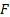is the payoff function: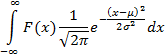Any payoff can be approximated arbitrarily accurately using combinations of piecewise polynomials. Even payoffs with discontinuities (e.g. digital options) can be approximated by combinations of value functions and (multiple) indicator pairs, where the pairs define the domain over which the relevant payoff function applies.

However, it is important to realise that prior to the representation of the pricing problem in the above format there is a transformation of variables that complicates how in practice this integral should best be evaluated.

The partial differential equation satisfied by any derivative in a Black-Scholes world is: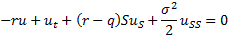We can convert this equation into a standard form with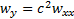using the following transformations: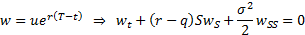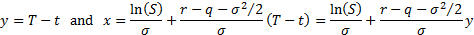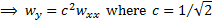To value European style payoffs we need to solve an initial value or Cauchy problem. If the boundary condition is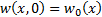at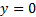, where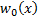is continuous and bounded for all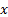then the solution becomes: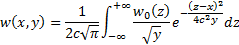Suppose that the payoff has the following general form: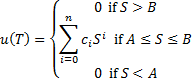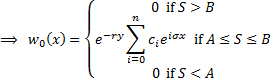The solution to the pricing problem is thus: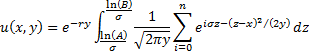The original polynomial elements in the payoff function are thus transformed into exponential terms in final integral representation.